Courses

# EMF & Torque Equation Electrical Engineering (EE) Notes | EduRev

## Electrical Engineering (EE) : EMF & Torque Equation Electrical Engineering (EE) Notes | EduRev

The document EMF & Torque Equation Electrical Engineering (EE) Notes | EduRev is a part of the Electrical Engineering (EE) Course Basic Electrical Technology.
All you need of Electrical Engineering (EE) at this link: Electrical Engineering (EE)

Introduction

Be it motor or generator operations, the analysis of D.C machine performance center around two fundamental equations namely the emf equation and the torque equations. In fact both motoring and generating actions go together in d.c machines. For example in a d.c motor there will be induced voltage across the brushes in the same way as in a generator. The induced voltage in d.c motor is however called by a different name back emf. Thus the factors on which induced emf in generator depend will be no different from motor action. In fact the same emf equation can be employed to calculate induced emf for both generator and motor operation. In the same way same torque equation can be used to calculate electromagnetic torque developed in both motoring and generating actions.

In this lesson these two fundamental equations have been derived.  Field patterns along the air gap of the machine for both motor and generator modes are explained. The ill effects of armature mmf (for a loaded machine) is discussed and possible remedial measures are presented. Calculation of cross magnetizing and demagnetizing AT (ampere turns) of d.c machines with shifted brush are presented. Depending on your course requirement the derivation for these ATs with sifted brush may be avoided. Finally, the phenomenon of armature reaction and a brief account of commutation are presented.

EMF & Torque Equations

In this section we shall derive two most fundamental and important formulas (namely emf and torque equations) for d.c machine in general. These will be extensively used to analyse the performance and to solve problems on d.c machines.

EMF Equation

Consider a D.C generator whose field coil is excited to produce a flux density distribution along the air gap and the armature is driven by a prime mover at constant speed as shown in figure 37.1.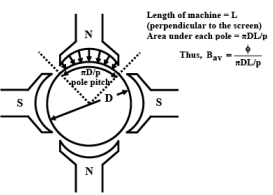Figure 37.1:  Pole pitch & area on armature surface per pole

Let us assume a p polar d.c generator is driven (by a prime mover) at n rps. The excitation of the stator field is such that it produces a φ Wb flux per pole. Also let z be the total number of armature conductors and a be the number of parallel paths in the armature circuit. In general, as discussed in the earlier section the magnitude of the voltage from one conductor to another is likely to very since flux density distribution is trapezoidal in nature. Therefore, total average voltage across the brushes is calculated on the basis of average flux density Bav. If D and L are the rotor diameter and the length of the machine in meters then area under each pole is ( πD/p ) L .

Hence average flux density in the gap is given by

Average flux density Bav  =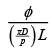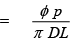Induced voltage in a single conductor  = BavLv

Number of conductors present in each parallel path  =Z/a

If v is the tangential veloc ity then, v = πDn

Therefore, total voltage appearing across the brushes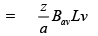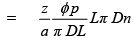Thus voltage induced across the armature, EA =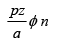We thus see that across the armature a voltage will be generated so long there exists some flux per pole and the machine runs with some speed. Therefore irrespective of the fact that the machine is operating as generator or as motor, armature has an induced voltage in it governed essentially by the above derived equation. This emf is called back emf for motor operation.

Torque equation

Whenever armature carries current in presence of flux, conductor experiences force which gives rise to the electromagnetic torque. In this section we shall derive an expression for the electromagnetic torque Te developed in a d.c machine. Obviously Te will be developed both in motor and generator mode of operation. It may be noted that the direction of conductor currents reverses as we move from one pole to the other. This ensures unidirectional torque to be produced. The derivation of the torque expression is shown below.

Let, I = Armature current

Average flux density Bav =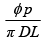Then, Ia /a  = Current flowing through each conductor.

Force on a single conductor  =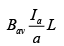Torque on a single conductor  =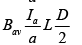Total electromagnetic torque developed, T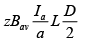Putting the value of Bav, we get Te =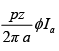Thus we see that the above equation is once again applicable both for motor and generator mode of operation. The direction of the electromagnetic torque, Te will be along the direction of rotation in case of motor operation and opposite to the direction of rotation in case of generator operation. When the machine runs steadily at a constant rpm then Te = Tload and Te = Tpm, respectively for motor and generator mode.

The emf and torque equations are extremely useful and should be remembered by heart.

GNP and MNP

In a unloaded d.c machine field is produced only by the field coil as armature does not carry any current. For a unloaded generator, net field is equal to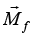produced by field coil alone and as shown in figure 37.2 (a). Then for a plane which is at right angles to, no field can exist along the plane, since Mf cos900 = 0. The plane along which there will be no field is called Magnetic Neutral Plane or MNP in short. The Geometrical Neutral Plane (GNP) is defined as a plane which is perpendicular to stator field axis. Thus for an unloaded generator GNP and MNP coincide. In a loaded generator, apart from, there will exist field produced by armature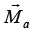as well making the resultant field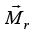shifted as shown in figure 37.2 (b). Thus MNP in this case will be perpendicular tor M . Therefore it may be concluded that MNP for generator mode gets shifted along the direction of rotation of the armature.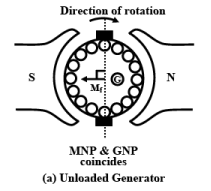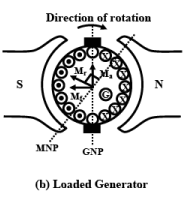Figure 37.2: MNP & GNP : Generator mode.

The shift of MNP for a loaded motor will be in a direction opposite to the rotation as depicted in figure 37.3 (b). The explanation of this is left to the reader as an exercise.

Armature reaction

In a unloaded d.c machine armature current is vanishingly small and the flux per pole is decided by the field current alone. The uniform distribution of the lines of force get upset when armature too carries current due to loading. In one half of the pole, flux lines are concentrated and in the other half they are rarefied. Qualitatively one can argue that during loading condition flux per pole will remain same as in no load operation because the increase of flux in one half will be balanced by the decrease in the flux in the other half. Since it is the flux per pole which decides the emf generated and the torque produced by the machine, seemingly there will be no effect felt so far as the performance of the machine is concerned due to armature reaction. This in fact is almost true when the machine is lightly or moderately loaded.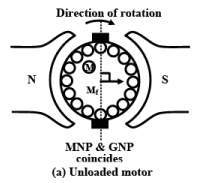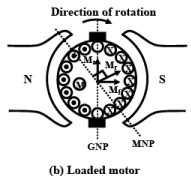Figure 37.3: MNP & GNP : Motor mode.

However at rated armature current the increase of flux in one half of the pole is rather less than the decrease in the other half due to presence of saturation. In other words there will be a net decrease in flux per pole during sufficient loading of the machine. This will have a direct bearing on the emf as well as torque developed affecting the performance of the machine.

Apart from this, due to distortion in the flux distribution, there will be some amount of flux present along the q-axis (brush axis) of the machine. This causes commutation difficult. In the following sections we try to explain armature reaction in somewhat detail considering motor and generator mode separately.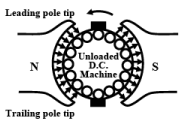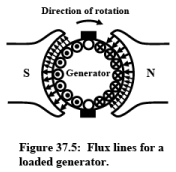Figure 37.4: Flux lines during no load condition.                 Figure 37.5:  Flux lines for a loaded generator.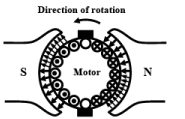Figure 37.6:  Flux lines for a loaded motor.

When a d.c machine operates absolutely under no load condition, armature current is zero. Under such a condition Te developed is zero and runs at constant no load speed. In absence of any Ia, the flux per pole φ, inside the machine is solely decided by the field current and lines of force are uniformly distributed under a pole as shown in figure

A generator gets loaded when a resistance across the armature is connected and power is delivered to the resistance. The direction of the current in the conductor (either cross or dot) is decided by the fact that direction of Te will be opposite to the direction of rotation. It is therefore obvious to see that flux per pole φ, developed in the generator should be decided not only by the mmf of the field winding alone but the armature mmf as well as the armature is carrying current now. By superposing the no load field lines and the armature field lines one can get the resultant field lines pattern as shown in Figures 37.5 and 37.7. The tip of the pole which is seen by a moving conductor first during the course of rotation is called the leading pole tip and the tip of the pole which is seen later is called the trailing pole tip. In case of generator mode we see that the lines of forces are concentrated near the trailing edge thereby producing torque in the opposite direction of rotation. How the trapezoidal no load field gets distorted along the air gap of the generator is shown in the Figure 37.7.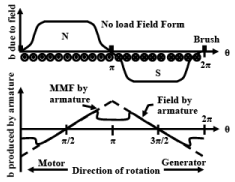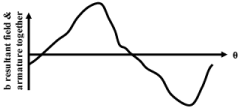Figure 37.7:  Effect of Armature Reaction

In this figure note that the armature mmf distribution is triangular in nature and the flux density distribution due to armature current is obtained by dividing armature mmf with the reluctance of the air gap. The reluctance is constant and small at any point under the pole. This means that the armature flux density will simply follow the armature mmf pattern. However, the reluctance in the q-axis region is quite large giving rise to small resultant flux of polarity same as the main pole behind in the q-axis.

In the same way one can explain the effect of loading a d.c motor by referring to Figures 37.6 and 37.7. Point to be noted here is that the lines of forces gets concentrated near the leading pole tip and rarefied near the trailing pole thereby producing torque along the direction of rotation. Also note the presence of some flux in the q-axis with a polarity same as main pole ahead.

Cross magnetising & Demagnetising AT

Usually the brushes in a d.c machine are along the GNP.  The armature mmfwhich acts always along the direction of the brush axis also acts along GNP.  It may also be noted thatis at right angles to the field mmfwhen brushes are not shifted.  Thushas cross magnetising effect on.Apparentlydoes not have any component opposingdirectly.  The presence of cross magnetising armature mmfdistorts the no load field pattern caused byThe cross magnetising armature AT can be calculated as shown below.

Let, P = Number of poles

z = Total number of armature conductors

a = Number of parallel paths

Armature current = Ia

Current through armature conductor = Ia / a

Total Ampere conductors =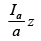Total AT  =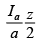∴Armature AT/pole =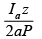Demagnetising by armature mmf can occur when a deliberate brush shift is introduced. Small brush shift is sometimes given to improve commutation.  For generator brush shift is given in the forward direction (in the direction of rotation) while for motor mode the brush shift is given in the backward direction (opposite to the direction of rotation) as shown in figures 37.8 and 37.9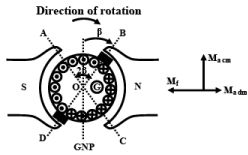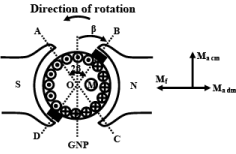Brush shift in forward direction for Generator                    Brush shift in backward direction for Motor

Figure 37.8:                                                                                        Figure 37.9:

Let the brush shift be β° (mechanical) for all the brushes.  Then as depicted in the figure 37.8 the conductors present within the angle 2 β° (i.e., ∠AOB and ∠COD) will be responsible for demagnetization and conductors present within the angle (180° - 2 β°) (i.e., ∠AOD and ∠BOC) will be responsible for crossmagnetisation for a 2 polar machine.  Ampere turns for demagnetization can be calculated as follows:

Number of conductors spread over 360° = z

Number of conductors spread over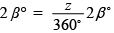Demagnetizing Ampere conductors contributed by 2 β° =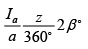Since brushes are placed in the inter polar regions and there are P number of brush positions,

∴Total number of conductors responsible for demagnetization =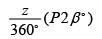Total Demagnetising Ampere conductors  =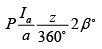Total Demagnetising Ampere turns =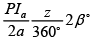Total Demagnetising Ampere turns per pole  =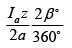To find expression for the cross magnetising, replace 2 β° by (180° - 2 β°) in the above expression to get:

Number of conductors responsible for cross magnetization =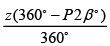Total cross Ampere turns  =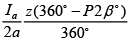Total cross Ampere turns per pole =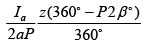It may easily be verified that the sum of demagnetizing AT/pole and cross magnetising AT/pole is equal to total AT/pole as shown below: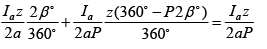Commutation & Armature reaction

If we concentrate our attention to a single conductor, we immediately recognize that the direction of current reverses as it moves from the influence of one pole to the influence of the next opposite pole.  This reversal of current in the conductor is called commutation.  During no load operation when the conductor reaches the magnetic neutral axis or the q-axis, the induced voltage in it is zero as there is no flux is present in the q-axis.  Also any coil present in this position and under going commutation, will get short circuited by the commutator segments and brushes.  In other words we see that every coil will be short circuited whenever it undergoes commutation and fortunately at that time induced emf in the coil being zero, no circulating current will be present at least during no load condition.  But as discussed earlier, flux in the quadrature axis will never be zero when the machine is loaded.  Hence coil undergoing commutation will have circulating current causing problem so far as smooth commutation is concerned.

For small machines (up to few kilo watts) no special care is taken to avoid the armature reaction effects.  However for large machines, to get rid of the ill effects of armature reaction one can use compensating winding, inter poles or both.

The basic idea of nullifying armature mmf is based on a very simple fact.  We know that a magnetic field is produced in the vicinity when a conductor carries current.  Naturally another conductor carrying same current but in the opposite direction if placed in close proximity of the first conductor, the resultant field in the vicinity will be close to zero.  Additional winding called compensating winding is placed on the pole faces of the machine and connected in series with the armature circuit in such a way that the direction of current in compensating winding is opposite to that in the armature conductor as shown in Figure 37.10.  It may be noted that compensating winding can not nullify the quadrature axis armature flux completely.

Additional small poles called inter poles are provided in between the main poles in large machines to get rid of the commutation problem arising out of armature reaction.   Sectional view of a machine provided with both compensating and inter poles is shown in Figure 37.11 and the schematic representation of such a machine is shown in Figure 37.12.

Careful inspection of the figures mentioned reveal that the polarity of the inter pole should be same as that of the main pole ahead in case of generator and should be same as that of main pole behind in case of motor.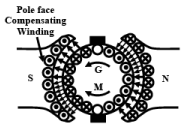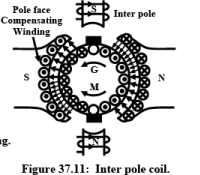Figure 37.10:  Position of compensating winding.                    Figure 37.11:  Inter pole coil.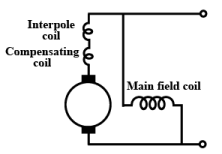Figure 37.12:  Interpole & compensating coil connection.

Offer running on EduRev: Apply code STAYHOME200 to get INR 200 off on our premium plan EduRev Infinity!

,

,

,

,

,

,

,

,

,

,

,

,

,

,

,

,

,

,

,

,

,

;